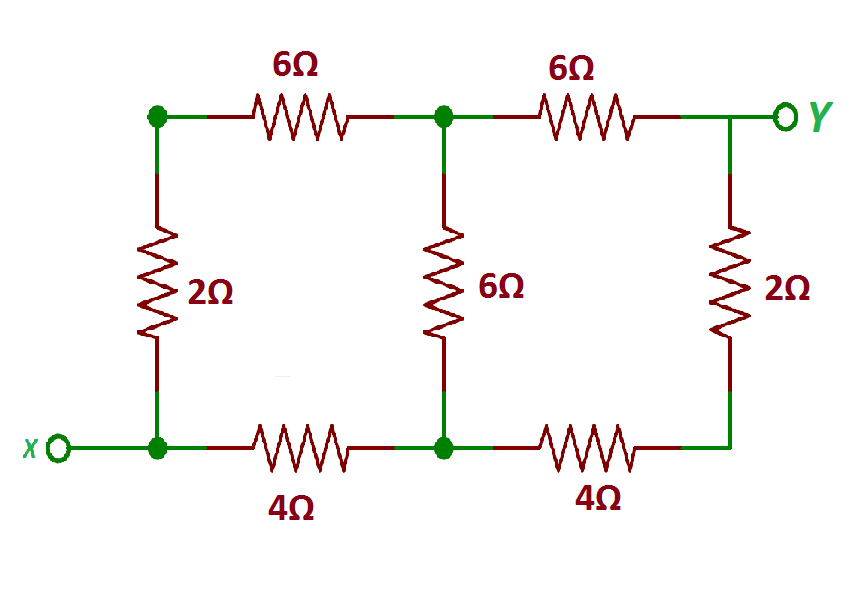MORE IN Basic Electrical Engineering (BEE)
SPPU First Year Engineering (Semester 1)
Basic Electrical Engineering (BEE)
December 2013
Total marks: --
Total time: --
INSTRUCTIONS
(1) Assume appropriate data and state your reasons
(2) Marks are given to the right of every question
(3) Draw neat diagrams wherever necessary

Answer any one question from Q1 & Q2
1 (a) Define Insulation Resistance and derive expression for it of a Cable.
6 M
1 (b) A single core cable has its conductor diameter as 2 cm and outer diameter as 5 cm. The resistivity of conductor and insulator are 1.73 ? 10-8 ? -m and 8?1012? -m respectively. Calculate resistance of conductor and insulation for a cable of 100 meter.
6 M

2 (a) Derive an expression for Energy stored in a magnetic field.
6 M
2 (b) An iron ring of mean circum ference of 50 cm has an airgap of 2mm cut in it. It has circular cross section with area of 5 cm2. It carries a coil wound with 600 turns wound uniformly. The relative permeability of iron is 580. If the coil carries a current of 2 amp. Find the Flux in air gap.
6 M

Answer any one question from Q3 & Q4
3 (a) Derive an expression of Average value of an alternating simusoidal current.
6 M
3 (b) An inductive coil, having negligible resistance and 0.1 H inductance, is connected across a 200V, 50Hz supply. Find
(i) Inductive reactance.
(ii) RMS value of current drawn. State equations for voltage & current.
6 M

4 (a) Compare core type and shell type transformer construction.
6 M
4 (b) A 25k VA, 2200/20V 50Hz single phase transformer has a primary resistance of 1.8 ? and secondary resistance of 0.02 ?. Calculate the transformer efficiency at half load 0.8 lagging p.f. The Iron Losses are 1000 watt.
6 M

Answer any one question from Q5 & Q6
5 (a) A circuit, consisting of resistance of 20 ? and inductance of 0.1 H in series, is connected across single phase 220V, 50Hz supply.
Calculate :
(1) Current drawn
(2) p.f. and
(3) Power consumed by ckt.
7 M
5 (b) Define:
(1) Impedance and
6 M

6 (a) Three coils each of 5? resistance and 6? inductive reactance are connected in Delta across 3 phase, 440V, 50Hz, A.C. Supply. Calculate current drawn p.f. of system and power consumed by circuit.
7 M
6 (b) A coil of 2? resistance and 0.01 H inductance is connected in series with a capacitor across 200V supply. What must be capacitance in order that maximum current is drawn at frequency of 50 Hz. Find also voltage across capacitance.
6 M

Answer any one question from Q7 & Q8
7 (a) State and explain kirchhoff?s laws.
8 M
7 (b) Calculate the equivalent resistance between terminals X and Y for the circuit shown in Fig, 15 M

8 (a) Apply Thevenin's theorem to calculate current flowing in branch A-B as shown in Fig. 2
8 M
8 (b) State and explain Superposition Theorem.
5 M

More question papers from Basic Electrical Engineering (BEE)